# Matlab-Matrix - Quick Guide

#### Data Preprocessing for Machine Learning using MATLAB

30 Lectures 4 hours

#### Image Processing Toolbox in MATLAB

17 Lectures 3 hours

#### Matlab - The Complete Course

Best Seller

37 Lectures 5 hours

# Matlab-Matrix - Introduction

MATLAB (matrix laboratory) is a fourth-generation high-level programming language and interactive environment for numerical computation, visualization and programming. It allows matrix manipulations; plotting of functions and data; implementation of algorithms; creation of user interfaces; interfacing with programs written in other languages, including C, C++, Java, and FORTRAN; analyze data; develop algorithms; and create models and applications.

In this tutorial we will focus on Matrix Implementation using MATLAB.

## Matrix

A matrix is a collection of numbers arranged in rows and columns that represents a rectangular array.

An example of matrix with 2 rows and 3 columns is as shown below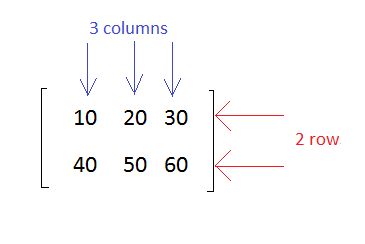## Matrix Dimension

The dimension of a matrix is defined based on the number of rows and columns.

A matrix with 2 rows and 3 columns is said to be 2x3 matrix.

A matrix with 3 rows and 3 columns is said to be 3x3 matrix.

## Matrix in Matlab

In MATLAB, you create a matrix by entering elements in each row as comma or space delimited numbers and using semicolons to mark the end of each row.

### Example

To create a 4x5 matrix, enter the following.

```a = [ 1 2 3 4 5; 2 3 4 5 6; 3 4 5 6 7; 4 5 6 7 8]
```

The matrix has 4 rows and 5 columns.

The first row will have values as 1 2 3 4 5

The second row: 2 3 4 5 6

The third row: 3 4 5 6 7

The fourth row: 4 5 6 7 8

### Output

The matrix of size 4x5 will look as follows

```a =
1  2  3  4  5
2  3  4  5  6
3  4  5  6  7
4  5  6  7  8
```

Let us test the matrix creation in MATLAB command window as shown below −

```>> a = [ 1 2 3 4 5; 2 3 4 5 6; 3 4 5 6 7; 4 5 6 7 8]

a =
1  2  3  4  5
2  3  4  5  6
3  4  5  6  7
4  5  6  7  8

>>
```

## Referencing the Elements

To reference an element in the mth row and nth column, of a matrix mx, we write the following

```mx(m, n);
```

## Example

To refer to the element in the 2nd row and 5th column, of the matrix a, as created in the last section, we type the following.

```>> a = [ 1 2 3 4 5; 2 3 4 5 6; 3 4 5 6 7; 4 5 6 7 8]

a =
1  2  3  4  5
2  3  4  5  6
3  4  5  6  7
4  5  6  7  8

>> a(2,5)

ans =
6

>>
```

To get all the elements of the nth column in a matrix , you can make use of A (:,n) where n represents the column no in the matrix.

```A(:,n).
```

### Example

Now, let us create a column vector v, from all the elements of the 4th column of the matrix a. This will be as follows

```a = [ 1 2 3 4 5; 2 3 4 5 6; 3 4 5 6 7; 4 5 6 7 8];
v = a(:,4)
```

### Output

MATLAB will execute the above statement and return the following result.

```>> a = [ 1 2 3 4 5; 2 3 4 5 6; 3 4 5 6 7; 4 5 6 7 8]

a =
1  2  3  4  5
2  3  4  5  6
3  4  5  6  7
4  5  6  7  8

>> v=a(:,4)

v =
4
5
6
7

>>
```

You can also select the elements in the mth through nth columns. For this, we write as follows.

```a(:,m:n)
```

### Example

Let us create a smaller matrix by taking the elements from the second and third columns, as shown below −

```a = [ 1 2 3 4 5; 2 3 4 5 6; 3 4 5 6 7; 4 5 6 7 8];
a(:, 2:3)
```

### Output

MATLAB will execute the above statement and return the following result −

```>> a = [ 1 2 3 4 5; 2 3 4 5 6; 3 4 5 6 7; 4 5 6 7 8]

a =
1  2  3  4  5
2  3  4  5  6
3  4  5  6  7
4  5  6  7  8

>> a(:, 2:3)

ans =
2  3
3  4
4  5
5  6

>>
```

In the same way, you can create a sub-matrix by taking a sub-part of a matrix.

## Example

Let us create a sub-matrix saby taking the inner subpart of a, as given below −

```3  4  5
4  5  6
```

During execution in MATLAB command window, the matrix will be as shown below −

```>> a = [ 1 2 3 4 5; 2 3 4 5 6; 3 4 5 6 7; 4 5 6 7 8]

a =
1  2  3  4  5
2  3  4  5  6
3  4  5  6  7
4  5  6  7  8

>> sa = a(2:3,2:4)

sa =
3  4  5
4  5  6

>>
```

# Matlab-Matrix - Environment Setup

The official website of MATLAB is https://www.mathworks.com.

The following page will appear on your screen −MATLAB is not free to download and you need to pay for the licensed copy. Later on you can download it.A free trial version is available for which you have to create a login for your respective account. Once an account is created, they allow you to download MATLAB and also an online version for a trial of 30 days’ license.Once you are done with the creating a login from their website, download MATLAB and install on your system. Then, start MATLAB or you can also make use of their online version that will be available once you sign in.

This is how the UI interface of MATLAB looks like when you install matlab or hit the online link of MATLAB.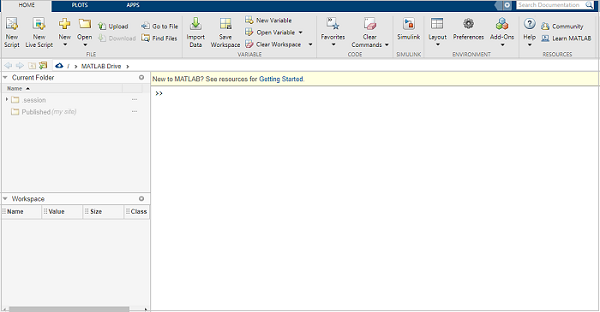## Understanding the MATLAB Environment

MATLAB development IDE can be launched from the icon created on the desktop. The main working window in MATLAB is called the desktop. When MATLAB is started, the desktop appears in its default layout.

As I said earlier if you are using the trial version can make use of the online link of MATLAB to get the IDE as shown below −Let us understand the MATLAB IDE.

## Current Folder

This panel allows you to access the project folders and files.## Command Window

This is the main area where commands can be entered at the command line. It is indicated by the command prompt (>>).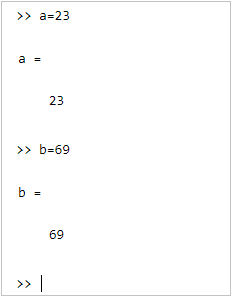## Workspace

The workspace shows all the variables created and/or imported from files.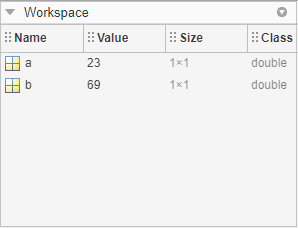# Matlab-Matrix - Create a Matrix

In MATLAB, you can create a matrix by entering the elements in each row as comma. You can also create a matrix with space delimited numbers and by using the semicolons to mark the end of each row.

## Matrix with single row

Let us create a simple matrix in MATLAB that has a single row and three elements. Each element should have a space or comma.

### Example

Consider the below mentioned elements to create a matrix.

```m=[2, 4, 6]
```

### Output

On execution in MATLAB it will display the following −

```>>m = [2, 4, 6]

m =

2  4  6
>>
```

When you execute the code in MATLAB, the result of the matrix is displayed in the command window.

## Matrix with Multiple rows

### Example

Let us now create a matrix with multiple rows. To do that, we need to separate each row with semicolon (;) as shown below −

```m = [2 4 6; 3 6 9; 4 8 12]
```

### Output

Here 2 4 6 is the first row, 3 6 9 is the second row and 4 8 12 is the third row. The matrix will be as follows −

```m = 2  4  6
3  6  9
4  8 12
```

Let us now execute the same in MATLAB command prompt, as mentioned below −

```>> m = [2 4 6; 3 6 9; 4 8 12]

m =

2  4  6
3  6  9
4  8 12

>>
```

The 3x3 matrix is displayed as shown above in MATLAB.

Besides creating matrix with the values of your choice you can also make use of the built-in MATLAB functions zeros, rand or ones to create a matrix as shown below −

### The zerosfunctions

This will create matrix with all zeros with the given row/column size.

Example

You can use MATLAB zeros function as follows −

```m0 = zeros(3,3)
```

Output

You will get the following output −

```>> m0 = zeros(3,3)

m0 =

0  0  0
0  0  0
0  0  0

>>
```

### The onesfunction

The matrix created will have ones as the values.

Example

You can use MATLAB ones function as follows −

```m1 = ones(3,3)
```

Output

You will get the following output −

```>> m1 = ones(3,3)

m1 =
1  1  1
1  1  1
1  1  1

>>
```

### The rand() function

The function rand() allows you to create a matrix with random elements for the size given. Here is an example for the same.

Example

```m1 = rand(3,3)
```

Output

Let us now execute the same in MATLAB to see the results. The output is as follows −

```>> m1 = rand(3,3)

m1 =

0.8147  0.9134  0.2785
0.9058  0.6324  0.5469
0.1270  0.0975  0.9575

>>
```

# Matlab-Matrix - Working with Matrices

In this chapter, I will deal with how to run the matrix inside MATLAB Environment to get the output. To define a matrix, and other matrix operations are discussed in details in the next chapters.

To get the matrix output in MATLAB will make use of −

• Command Prompt
• Using m-file

## Using Command Prompt

You can execute matrices directly inside command prompt. Here is an example wherein we have two matrices a and b.

The operation a+b gives the sum of matrix a and b.

The operation a-b gives the subtraction of matrix a and b.

The output is command prompt is as shown below −

```>> a = [ 1 2 3 ; 4 5 6; 7 8 9];
>> b = [ 7 5 6 ; 2 0 8; 5 7 1];
>> c = a + b

c =

8   7   9
6   5  14
12  15  10

>> d = a - b

d =
-6  -3  -3
2   5  -2
2   1   8

>>
```

## Using m-file

You can also make use of a file to write the code and later execute it inside command prompt as shown below −

Click on New script as shown below −This is open a new unsaved file as shown below −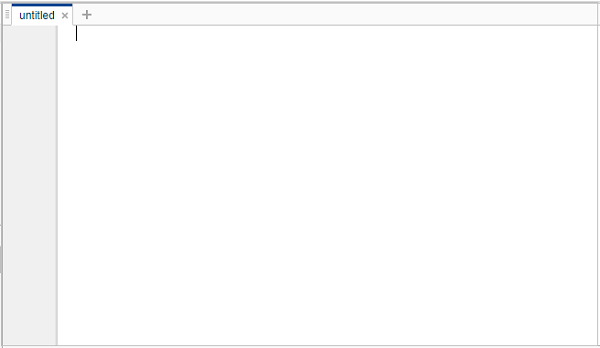Save the file and write your code insideThe file is saved as testmatrix.m.

Now you can use the run button or type the name of the file inside command window.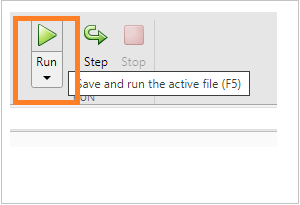The output will be shown in the command window as shown below −

```>> testmatrix

c =
8   7   9
6   5  14
12  15  10

d =
-6  -3  -3
2   5  -2
2   1   8

>>
```

# Matlab-Matrix - Multiplication

Consider two matrices A and B. If A is an m x n matrix and B is an n x p matrix, they could be multiplied together to produce an m x n matrix C. Matrix multiplication is possible only if the number of columns n in A is equal to the number of rows n in B.

In matrix multiplication, the elements of the rows in the first matrix are multiplied with the corresponding columns in the second matrix.

Each element in the (i, j)thposition, in the resulting matrix C, is the summation of the products of elements in ith row of the first matrix with the corresponding element in the jth column of the second matrix.

Matrix multiplication in MATLAB is performed by using the * operator.

### Example

Consider following example in MATLAB

```a = [ 1 2 3; 2 3 4; 1 2 5];
b = [ 2 1 3 ; 5 0 -2; 2 3 -1];
prod = a * b
```

### Output

The execution in MATLAB will display the following result −

```>> a = [ 1 2 3; 2 3 4; 1 2 5];
b = [ 2 1 3 ; 5 0 -2; 2 3 -1];
prod = a * b

prod =

18  10  -4
27  14  -4
22  16  -6

>>
```

## The mtimes function

You can also make use of the function mtimes to multiply two given matrices. It is a builtin function available in MATLAB.

### Example

Consider following example −

```a = [ 1 2 3; 2 3 4; 1 2 5];
b = [ 2 1 3 ; 5 0 -2; 2 3 -1];
test= mtimes(a,b)
```

### Output

On execution in MATLAB the output is as follows −

```>> a = [ 1 2 3; 2 3 4; 1 2 5];
b = [ 2 1 3 ; 5 0 -2; 2 3 -1];
test= mtimes(a,b)

test =

18  10  -4
27  14  -4
22  16  -6

>>
```

# Matlab-Matrix - Addition

To add two matrices, both the operand matrices must have the same number of rows and columns.

### Example

Here is an example

```a = [ 1 2 3 ; 4 5 6; 7 8 9];
b = [ 7 5 6 ; 2 0 8; 5 7 1];
c = a + b
```

### Output

On execution in MATLAB the result is as follows −

```>> a = [ 1 2 3 ; 4 5 6; 7 8 9];
b = [ 7 5 6 ; 2 0 8; 5 7 1];
c = a + b

c =

8  7   9
6  5  14
12 15  10

>>
```

## The plus() function

You can also make use of plus() built-in function to add two matrices as shown below −

### Example

Consider the following example for the use of plus() function to add the two matrices −

```a = [ 1 2 3 ; 4 5 6; 7 8 9];
b = [ 7 5 6 ; 2 0 8; 5 7 1];
c = plus(a,b)
```

### Output

The execution in MATLAB is as shown below −

```>> a = [ 1 2 3 ; 4 5 6; 7 8 9];
b = [ 7 5 6 ; 2 0 8; 5 7 1];
c = plus(a,b)

c =

8   7   9
6   5  14
12  15  10

>>
```

# Matlab-Matrix - Subtraction

To subtract two matrices, both the operand matrices must have the same number of rows and columns.

### Example

Here is an example −

```a = [ 1 2 3 ; 4 5 6; 7 8 9];
b = [ 7 5 6 ; 2 0 8; 5 7 1];
c = a - b
```

### Output

On execution in MATLAB the result is as follows −

```>> a = [ 1 2 3 ; 4 5 6; 7 8 9];
b = [ 7 5 6 ; 2 0 8; 5 7 1];
c = a - b

c =

-6  -3  -3
2   5  -2
2   1   8

>>
```

## The minus() Function

You can also make use of the minus() built-in function to subtract two matrices.

### Example

Consider the following example for use of minus() function for subtraction of two matrices −

```a = [ 1 2 3 ; 4 5 6; 7 8 9];
b = [ 7 5 6 ; 2 0 8; 5 7 1];
c = minus(a , b)
```

### Output

You will get the following result −

```>> a = [ 1 2 3 ; 4 5 6; 7 8 9];
b = [ 7 5 6 ; 2 0 8; 5 7 1];
c = minus(a , b)

c =

-6  -3  -3
2   5  -2
2   1   8

>>
```

# Matlab-Matrix - Determinant

Determinant of a matrix is calculated by using the det function of MATLAB. For example, the determinant of a matrix A is given by det(A).

## Example

Consider following example for calculating the determinant of a matrix −

```a = [ 1 2 3; 2 3 4; 1 2 5];
test = det(a)
```

## Output

The code on execution in MATLAB is as follows −

```>> a = [ 1 2 3; 2 3 4; 1 2 5];
test = det(a)

test =

-2
>>
```

# Matlab-Matrix - Inverse

The inverse of a matrix A is denoted by A−1 such that the following relationship holds −

```AA−1 = A−1A = 1
```

The inverse of a matrix does not always exist. If the determinant of the matrix is zero, then the inverse does not exist and the matrix is singular.

Inverse of a matrix in MATLAB is calculated using the inv function. Inverse of a matrix A is given by inv(A).

## Example

Here is an example to calculate inverse of given matrix −

```a = [ 1 2 3; 2 3 4; 1 2 5];
test = inv(a)
```

## Output

The execution in MATLAB gives following result −

```>> a = [ 1 2 3; 2 3 4; 1 2 5];
test = inv(a)

test =

-3.5000   2.0000   0.5000
3.0000  -1.0000  -1.0000
-0.5000       0    0.5000

>>
```

# Matlab-Matrix - Trace

Trace helps you to calculate the sum of diagonal elements in a given matrix.

## Example

Consider the given 3x3 matrix. Let us find out the sum of diagonal elements as shown below −

```a = [ 1 2 3; 2 3 4; 1 2 5];
test = trace(a)
```

## Output

The execution in MATLAB is as follows −

```>> a = [ 1 2 3; 2 3 4; 1 2 5]
test = trace(a)

a =

1  2  3
2  3  4
1  2  5

test =

9

>>
```

# Matlab-Matrix - Rank

The rank of the matrix is the number of linearly independent columns in a matrix. The function rank() helps to return the rank of a given matrix.

## Example

Consider following example for the use of rank() function for a matrix −

```a = [ 1 2 3; 2 3 4; 1 2 5]
test = rank(a)
```

## Output

The output in MATLAB on execution of the code is as follows −

```>> a = [ 1 2 3; 2 3 4; 1 2 5]
test = rank(a)

a =

1  2  3
2  3  4
1  2  5

test =

3

>>
```

# Matlab-Matrix - Transpose

The transpose operation switches the rows and columns in a matrix. It is represented by a single quote(').

### Example

Consider following example −

```a = [ 10 12 23 ; 14 8 6; 27 8 9]
b = a'
```

### Output

The execution in MATLAB gives the following output −

```>> a = [ 10 12 23 ; 14 8 6; 27 8 9]
b = a'

a =

10  12  23
14   8   6
27   8   9

b =

10  14  27
12   8   8
23   6   9

>>
```

## The transpose() function

You can also make use of the transpose() function to get the transpose of a matrix.

### Example

Consider the following example for use of transpose() function −

```a = [ 10 12 23 ; 14 8 6; 27 8 9]
b = transpose(a)
```

### Output

You will get the following output −

```>> a = [ 10 12 23 ; 14 8 6; 27 8 9]
b = transpose(a)

a =

10  12  23
14   8   6
27   8   9

b =

10  14  27
12   8   8
23   6   9

>>
```

# Matlab-Matrix - Deletion of Row & Column

You can delete an entire row or column of a matrix by assigning an empty set of square braces [] to that row or column. Basically, [] denotes an empty array.

## Example

For example, let us delete the fourth row of a, as shown below −

```a = [ 1 2 3 4 5; 2 3 4 5 6; 3 4 5 6 7; 4 5 6 7 8];
a( 4 , : ) = []
```

## Output

Here is the execution of above code in MATLAB

```>> a = [ 1 2 3 4 5; 2 3 4 5 6; 3 4 5 6 7; 4 5 6 7 8]
a( 4 , : ) = []

a =

1  2  3  4  5
2  3  4  5  6
3  4  5  6  7
4  5  6  7  8

a =

1  2  3  4  5
2  3  4  5  6
3  4  5  6  7

>>
```

The fourth row is deleted. It displays only three rows.

## Example

Next, let us delete the fifth column of a, as shown below −

```a = [ 1 2 3 4 5; 2 3 4 5 6; 3 4 5 6 7; 4 5 6 7 8]
a(: , 5)=[]
```

## Output

Let us see the execution of the above code in MATLAB −

```>> a = [ 1 2 3 4 5; 2 3 4 5 6; 3 4 5 6 7; 4 5 6 7 8]
a(: , 5)=[]

a =

1  2  3  4  5
2  3  4  5  6
3  4  5  6  7
4  5  6  7  8

a =

1  2  3  4
2  3  4  5
3  4  5  6
4  5  6  7

>>
```